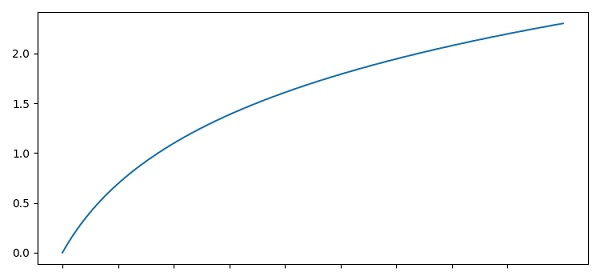# How to hide ticks label in Python but keep the ticks in place?

To hide ticks label and keep the ticks in place, we can take the following steps −

• Initialize x1 and x10 variables to get the x and y points, using numpy.

• Plot points x and y using the plot() method.

• Using xticks method, get or set the current tick locations and labels of the X-axis. Pass no arguments to return the current values without modifying them. So, pass the range(x1, x10) to get ticks but pass an empty list to hide the labels.

• To display the figure, use the show() method.

## Example

import numpy as np
from matplotlib import pyplot as plt
plt.rcParams["figure.figsize"] = [7.50, 3.50]
plt.rcParams["figure.autolayout"] = True
x1 = 1
x10 = 10
x = np.linspace(1, 10, 100)
y = np.log(x)
plt.plot(x, y)
plt.xticks(ticks=range(x1, x10), labels=[])
plt.show()

## Output